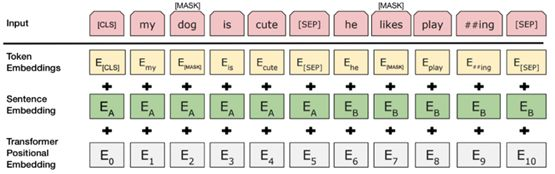Auto ByteScience AImedium来源李雷、睡不着的iris、Aileen编译

# 搞定NLP领域的“变形金刚”！手把手教你用BERT进行多标签文本分类

ELMo，ULMFiT 和OpenAI Transformer是其中几个关键的里程碑。所有这些算法都允许我们在大型数据库（例如所有维基百科文章）上预先训练无监督语言模型，然后在下游任务上对这些预先训练的模型进行微调。

### 从哪开始？

• BERT-Base, Multilingual Cased (最新推荐)：104种语言，12层，768个隐藏单元，自注意力的 head数为12，110M参数

### 数据展示

• text_a：文本评论

• text_b：未使用

• 标签：来自训练数据集的评论标签列表（很明显，测试数据集的标签将为空）

```class InputExample(object):
"""A single training/test example for sequence classification."""

def __init__(self, guid, text_a, text_b=None, labels=None):
"""Constructs a InputExample.

Args:
guid: Unique id for the example.
text_a: string. The untokenized text of the first sequence. For single
sequence tasks, only this sequence must be specified.
text_b: (Optional) string. The untokenized text of the second sequence.
Only must be specified for sequence pair tasks.
labels: (Optional) [string]. The label of the example. This should be
specified for train and dev examples, but not for test examples.
"""
self.guid = guid
self.text_a = text_a
self.text_b = text_b
self.labels = labels```
```class InputFeatures(object):
"""A single set of features of data."""

def __init__(self, input_ids, input_mask, segment_ids, label_ids):
self.input_ids = input_ids
self.segment_ids = segment_ids
self.label_ids = label_ids```• input_ids：标记化文本的数字id列表

• segment_ids：对于我们的情况，这将被设置为全1的列表

• label_ids：文本的one-hot编码标签

### 标记化(Tokenisation)

BERT-Base，uncased模型使用包含30,522个单词的词汇表。标记化过程涉及将输入文本拆分为词汇表中可用的标记列表。为了处理不在词汇表中的单词，BERT使用一种称为基于双字节编码（BPE，Byte-Pair Encoding）的WordPiece标记化技术。

### 模型架构

```class BertForMultiLabelSequenceClassification(PreTrainedBertModel):
"""BERT model for classification.
This module is composed of the BERT model with a linear layer on top of
the pooled output.
"""
def __init__(self, config, num_labels=2):
super(BertForMultiLabelSequenceClassification, self).__init__(config)
self.num_labels = num_labels
self.bert = BertModel(config)
self.dropout = torch.nn.Dropout(config.hidden_dropout_prob)
self.classifier = torch.nn.Linear(config.hidden_size, num_labels)
self.apply(self.init_bert_weights)

def forward(self, input_ids, token_type_ids=None, attention_mask=None, labels=None):
_, pooled_output = self.bert(input_ids, token_type_ids, attention_mask, output_all_encoded_layers=False)
pooled_output = self.dropout(pooled_output)
logits = self.classifier(pooled_output)

if labels is not None:
loss_fct = BCEWithLogitsLoss()
loss = loss_fct(logits.view(-1, self.num_labels), labels.view(-1, self.num_labels))
return loss
else:
return logits

def freeze_bert_encoder(self):
for param in self.bert.parameters():

def unfreeze_bert_encoder(self):
for param in self.bert.parameters():

```BertForMultiLabelSequenceClassification(
(bert): BertModel(
(embeddings): BertEmbeddings(
(word_embeddings): Embedding(28996, 768)
(position_embeddings): Embedding(512, 768)
(token_type_embeddings): Embedding(2, 768)
(LayerNorm): FusedLayerNorm(torch.Size(), eps=1e-12, elementwise_affine=True)
(dropout): Dropout(p=0.1)
)
(encoder): BertEncoder(
(layer): ModuleList(
#       12 BertLayers
(11): BertLayer(
(attention): BertAttention(
(self): BertSelfAttention(
(query): Linear(in_features=768, out_features=768, bias=True)
(key): Linear(in_features=768, out_features=768, bias=True)
(value): Linear(in_features=768, out_features=768, bias=True)
(dropout): Dropout(p=0.1)
)
(output): BertSelfOutput(
(dense): Linear(in_features=768, out_features=768, bias=True)
(LayerNorm): FusedLayerNorm(torch.Size(), eps=1e-12, elementwise_affine=True)
(dropout): Dropout(p=0.1)
)
)
(intermediate): BertIntermediate(
(dense): Linear(in_features=768, out_features=3072, bias=True)
)
(output): BertOutput(
(dense): Linear(in_features=3072, out_features=768, bias=True)
(LayerNorm): FusedLayerNorm(torch.Size(), eps=1e-12, elementwise_affine=True)
(dropout): Dropout(p=0.1)
)
)
)
)
(pooler): BertPooler(
(dense): Linear(in_features=768, out_features=768, bias=True)
(activation): Tanh()
)
)
(dropout): Dropout(p=0.1)
(classifier): Linear(in_features=768, out_features=6, bias=True)
)```
• BertEmbeddings:输入嵌入层

• BertEncoder: 12个BERT模型attention层

• 分类器：我们的多标签分类器，out_features = 6，每个分类符对应6个标签

### 评估指标

```def accuracy_thresh(y_pred:Tensor, y_true:Tensor, thresh:float=0.5, sigmoid:bool=True):
"Compute accuracy when `y_pred` and `y_true` are the same size."
if sigmoid: y_pred = y_pred.sigmoid()

return np.mean(((y_pred>thresh)==y_true.byte()).float().cpu().numpy(), axis=1).sum()```
```from sklearn.metrics import roc_curve, auc

# Compute ROC curve and ROC area for each class
fpr = dict()
tpr = dict()
roc_auc = dict()

for i in range(num_labels):
fpr[i], tpr[i], _ = roc_curve(all_labels[:, i], all_logits[:, i])
roc_auc[i] = auc(fpr[i], tpr[i])

# Compute micro-average ROC curve and ROC area
fpr["micro"], tpr["micro"], _ = roc_curve(all_labels.ravel(), all_logits.ravel())
roc_auc["micro"] = auc(fpr["micro"], tpr["micro"])```

### 评估分数

• 普通恶评：0.9988

• 严重恶评：0.9935

• 污言秽语：0.9988

• 威胁：0.9989

• 侮辱：0.9975

• 身份仇视：0.9988

• 微观平均ROC-AUC得分：0.9987

### Kaggle竞赛结果### 结论和后续